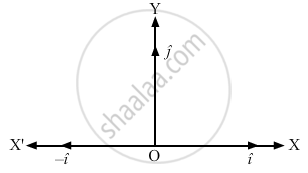Department of Pre-University Education, KarnatakaPUC Karnataka Science Class 11

# Can You Add Three Unit Vectors to Get a Unit Vector? Does Your Answer Change If Two Unit Vectors Are Along the Coordinate Axes? - Physics

Can you add three unit vectors to get a unit vector? Does your answer change if two unit vectors are along the coordinate axes?

#### Solution

Yes we can add three unit vectors to get a unit vector.
No, the answer does not change if two unit vectors are along the coordinate axes. Assume three unit vectors $\hat{i,} - \hat { i} \text { and }\hat { j}$ along the positive x-axis, negative x-axis and positive y-axis, respectively. Consider the figure given below:The magnitudes of the three unit vectors ( $\hat {i,} - \hat { i }\text { and } \hat {j})$ are the same, but their directions are different.
So, the resultant of $\hat { i} \text { and } - \hat {i}$ is a zero vector.
Now, $\hat {j} + \vec{0} = \hat {j}$    (Using the property of zero vector)
∴ The resultant of three unit vectors ( $\hat{i,} - \hat {i} \text { and }\hat { j }$)  is a unit vector ($\hat {j}$).

Concept: What is Physics?
Is there an error in this question or solution?

#### APPEARS IN

HC Verma Class 11, Class 12 Concepts of Physics Vol. 1
Chapter 2 Physics and Mathematics
Short Answers | Q 4 | Page 27
Share